# Rank statistic

(diff) ← Older revision | Latest revision (diff) | Newer revision → (diff)

A statistic (cf. Statistical estimator) constructed from a rank vector. If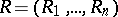is the rank vector constructed from a random observation vector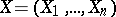, then any statisticwhich is a function ofis called a rank statistic. A classical example of a rank statistic is the Kendall coefficient of rank correlationbetween the vectorsand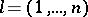, defined by the formula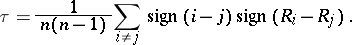In the class of all rank statistics a special place is occupied by so-called linear rank statistics, defined as follows. Let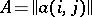be an arbitrary square matrix of order. Then the statistic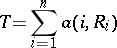is called a linear rank statistic. For example, the Spearman coefficient of rank correlation, defined by the formulais a linear rank statistic.

Linear rank statistics are, as a rule, simple to construct from the computational point of view and their distributions are easy to find. For this reason the notion of projection of a rank statistic into the family of linear rank statistics plays an important role in the theory of rank statistics. Ifis a rank statistic constructed from a random vectorunder a hypothesisabout its distribution, then a linear rank statistic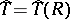such thatis minimal under the condition that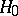is true, is called the projection ofinto the family of linear rank statistics. As a rule,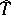approximateswell enough and the difference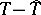is negligibly small as. If the hypothesisunder which the componentsof the random vectorare independent random variables is true, then the projectionofcan be determined by the formula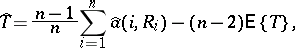(*)

where,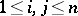(see ).

There is an intrinsic connection betweenand. It is shown in  that the projection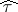of the Kendall coefficientinto the family of linear rank statistics coincides, up to a multiplicative constant, with the Spearman coefficient; namely,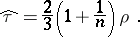This equality implies that the correlation coefficient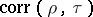betweenandis equal to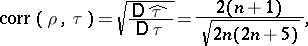implying that these rank statistics are asymptotically equivalent for large(cf. ).

How to Cite This Entry:
Rank statistic. Encyclopedia of Mathematics. URL: http://encyclopediaofmath.org/index.php?title=Rank_statistic&oldid=18903
This article was adapted from an original article by M.S. Nikulin (originator), which appeared in Encyclopedia of Mathematics - ISBN 1402006098. See original article